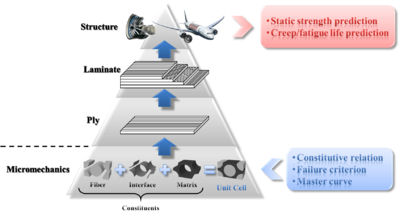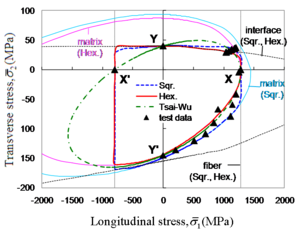# Micro-Mechanics of Failure

﻿
Micro-Mechanics of FailureFigure 1. Hierarchy of micromechanics-based analysis procedure for composite structures.

Micro-Mechanics of Failure (MMF) is a newly proposed methodology, providing a more logical explanation of failure mechanism of continuous fiber reinforced composites than other phenomenological models such as Tsai-Wu and Hashin failure criteria. The basic concept of MMF is to perform failure analysis of fiber reinforced composites, based upon micro stresses in each constituent (fiber, matrix, and fiber-matrix interface) calculated from macro stresses at the ply level. The hierarchy of micromechanics-based analysis procedure for composite structures is shown in Figure 1: starting from mechanical behavior of constituents, i.e., the fiber, the matrix, and the interface, the mechanical behavior of a ply, a laminate, and eventually a structure, can be predicted. At the constituent level, three elements are required to fully characterize each constituent:

• Constitutive relation, which describes the transient or time-independent response of the constituent to external mechanical as well as hygrothermal loadings;
• Master curve, which describes the time-dependent behavior of the constituent under creep or fatigue loadings;
• Failure criterion, which describes conditions that cause failure of the constituent.

The constituents and a unidirectional (UD) lamina are linked via a proper micromechanical model, so that ply properties can be derived from constituent properties, and on the other hand, micro stresses at the constituent level can be calculated from macro stresses at the ply level.

## Unit cell modelFigure 2. Schematic illustration of idealized fiber arrays and their corresponding unit cells.

Starting from the constituent level, it is necessary to devise a proper method to organize all three constituents such that the microstructure of a UD lamina is well-described. In reality, all fibers in a UD ply are aligned longitudinally; however, in the cross-sectional view, the distribution of fibers is random(Figure 2), and there is no distinguishable regular pattern in which fibers are arrayed. To avoid such a complication cause by the random arrangement of fibers, an idealization of the fiber arrangement in a UD lamina is performed, and the result is the regular fiber packing pattern. Two regular fiber packing patterns are considered: the square array and the hexagonal array (Figure 2). Either array can be viewed as a repetition of a single element, named unit cell or representative volume element (RVE), which consists of all three constituents. With periodical boundary conditions applied, a unit cell is able to respond to external loadings in the same way that the whole array does. Therefore, a unit cell model is sufficient in representing the microstructure of a UD ply.

## Stress amplification factor (SAF)

Stress distribution at the laminate level due to external loadings applied to the structure can be acquired using finite element analysis (FEA). Stresses at the ply level can be obtained through transformation of laminate stresses from laminate coordinate system to ply coordinate system. To further calculate micro stresses at the constituent level, the unit cell model is employed. Micro stresses$\boldsymbol{\sigma}$ at any point within fiber/matrix, and micro surface tractions$\mathbf{t}$ at any interfacial point, are related to ply stresses$\boldsymbol{\bar{\sigma}}$ as well as temperature increment ΔT through :$\begin{array}{lcl} \boldsymbol{\sigma}_\mathit{f}&=&\mathbf{M}_\mathit{f}\boldsymbol{\bar{\sigma}} + \mathbf{A}_\mathit{f}\Delta T\\ \boldsymbol{\sigma}_\mathit{m}&=&\mathbf{M}_\mathit{m}\boldsymbol{\bar{\sigma}} + \mathbf{A}_\mathit{m}\Delta T\\ \mathbf{t}_\mathit{i}&=&\mathbf{M}_\mathit{i}\boldsymbol{\bar{\sigma}} + \mathbf{A}_\mathit{i}\Delta T \end{array}$

(1)$\boldsymbol{\sigma}$,$\boldsymbol{\bar{\sigma}}$, and$\mathbf{t}$ are columm vectors with 6, 6, and 3 components, respectively. Subscripts serve as indications of constituents, i.e. f for fiber, m for matrix, and i for interface.$\mathbf{M}$ and$\mathbf{A}$ are respectively called stress amplification factors (SAF) for macro stresses and for temperature increment. The SAF serves as a conversion factor between macro stresses at the ply level and micro stresses at the constituent level. For a micro point in fiber or matrix,$\mathbf{M}$ is a 6×6 matrix while$\mathbf{A}$ has the dimension of 6×1; for an interfacial point, respective dimensions of$\mathbf{M}$ and$\mathbf{A}$ are 3×6 and 3×1. The value of each single term in the SAF for a micro material point is determined through FEA of the unit cell model under given macroscopic loading conditions. The definition of SAF is valid not only for constituents having linear elastic behavior and constant coefficients of thermal expansion (CTE), but also for those possessing complex constitutive relations and variable CTEs.

## Constituent failure criteria

### Fiber failure criterion

Fiber is taken as transversely isotropic, and there are two alternative failure criteria for it: a simple maximum stress criterion and a quadratic failure criterion extended from Tsai-Wu failure criterion:$\begin{array}{lcl} \text{Maximum stress failure criterion: }-\mathit{X}^\prime_\mathit{f} < \sigma_1 < \mathit{X}_\mathit{f}\\ \text{Quadratic failure criterion: }\displaystyle\sum_{\mathit{j}=1}^6\displaystyle\sum_{\mathit{i}=1}^6\mathit{F}_\mathit{ij}\sigma_\mathit{i}\sigma_\mathit{j} + \displaystyle\sum_{\mathit{i}=1}^6\mathit{F}_\mathit{i}\sigma_\mathit{i} = 1 \end{array}$

(2)

Stresses used in two preceding criteria should be micro stresses in the fiber, expressed in such a coordinate system that 1-direction signifies the longitudinal direction of fiber.

### Matrix failure criterion

The polymeric matrix is assumed to be isotropic and exhibits a higher strength under uniaxial compression than under uniaxial tension. A modified version of von Mises failure criterion suggested by Christensen is adopted for the matrix:$\begin{array}{lcl} \cfrac{\sigma_\mathit{Mises}^2}{\mathit{C}_\mathit{m}\mathit{T}_\mathit{m}} + \left(\cfrac{1}{\mathit{T}_\mathit{m}} - \cfrac{1}{\mathit{C}_\mathit{m}}\right)\mathit{I}_1 = 1 \end{array}$

(3)

Tm and Cm represent matrix tensile and compressive strength, respectively; whereas σMises and I1 are von Mises equivalent stress and the first stress invariant of micro stresses at a point within matrix, respectively.

### Interface failure criterion

The fiber-matrix interface features traction-separation bahavior, and the failure criterion dedicated to it takes the following form :$\begin{array}{lcl} \left(\cfrac{\left\langle\mathit{t}_\mathit{n}\right\rangle}{\mathit{Y}_\mathit{n}}\right)^2 + \left(\cfrac{\mathit{t}_\mathit{s}}{\mathit{Y}_\mathit{s}}\right)^2 = 1 \end{array}$

(4)

where tn and ts are normal (perpendicular to the interface) and shear (tangential to the interface) interfacial tractions, with Yn and Ys being their corresponding strengths. The angle brackets (Macaulay brackets) imply that a pure compressive normal traction does not contribute to interface failure.

## Comparison between MMF and other failure criteriaFigure 3. Comparison between theoretical failure predictions and test data.

As a completely mechanics-based failure theory, MMF outperforms other failure criteria in that it is able to distinguish the critical constituent in the critical ply in a composite laminate. The failure envelopes generated by MMF and Tsai-Wu failure criterion for a carbon/epoxy UD ply are plotted in Figure 3, with test data superimposed. Failed constituent can be directly observed from the failure envelope predicted by MMF, while the failure envelope predicted by Tsai-Wu is unable to yield such information.

## Further extension of MMF

Endeavors have been made to incorporate MMF with multiple progressive damage models and fatigue models for strength and life prediction of composite structures subjected to static or dynamic loadings.

Wikimedia Foundation. 2010.

### Look at other dictionaries:

• Micro Instrumentation and Telemetry Systems — MITS logo used from 1972 to 1975. Micro Instrumentation and Telemetry Systems (MITS) was an American electronics company founded in Albuquerque, New Mexico that began manufacturing electronic calculators in 1971 and personal computers in 1975. …   Wikipedia

• Christensen Failure Criterion — Failure criteria for isotropic materials has been a long standing problem. Despite many historical attempts, there have not been any successful general forms that span the range from ductile to brittle materials. A new failure criterion that aims …   Wikipedia

• Contact mechanics — Continuum mechanics …   Wikipedia

• GENOA Software — GENOA is an integrated engineering software suite by AlphaSTAR Corporation for virtual testing of material structures involving composites and metals. Integrating diverse structural analysis and design modules, it is known for cost effectiveness… …   Wikipedia

• Mechanical engineering — Mechanical engineers design and build engines and power plants …   Wikipedia

• Glossary of fuel cell terms — The Glossary of fuel cell terms lists the definitions of many terms used within the fuel cell industry. The terms in this glossary may be used by fuel cell industry associations, in education material and fuel cell codes and standards to name but …   Wikipedia

• Friction — For other uses, see Friction (disambiguation). Classical mechanics …   Wikipedia

• Ceramic materials — Ceramic Si3N4 bearing parts Ceramic materials are inorganic, non metallic materials and things made from them. They may be crystalline or partly crystalline. They are formed by the action of heat and subsequent cooling. Clay was one of the… …   Wikipedia

• Hardware random number generator — This SSL Accelerator computer card uses a hardware random number generator to generate cryptographic keys to encrypt data sent over computer networks. In computing, a hardware random number generator is an apparatus that generates random numbers… …   Wikipedia

• Europe, history of — Introduction       history of European peoples and cultures from prehistoric times to the present. Europe is a more ambiguous term than most geographic expressions. Its etymology is doubtful, as is the physical extent of the area it designates.… …   Universalium